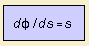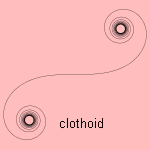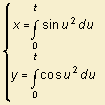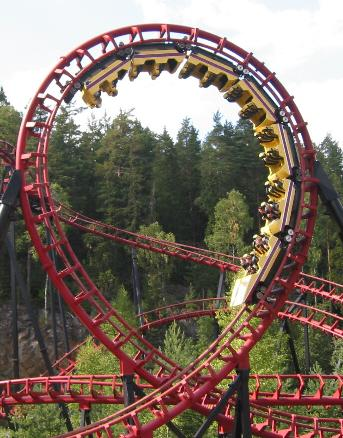# Euler's spiral

## spiralThe curvature of EulerÇs spiral is linearly related to its arc length 1). When the path of the curve is followed with an uniform velocity, the speed of rotation is linear (in time). That's why the curve with the reverse relation is called the anti-clothoid.Most authors present the curveÇs formula in a parametric form:The formulas under the integral can also be written as the Bessel functions J1/2 (for y) and J-1/2 (for x). The spiral,  the x and y component are both a Fresnel integral of a square root. It was the famous Leonhard Euler who investigated the curve as first (in 1744). Other names for the spiral are clothoid and spiral of Cornu or Cornu spiral. It was the French physicist Marie Alfred Cornu (1841-1902) who used the spiral to describe diffraction from the edge of a half-plane. In the early days of  railways it was perfectly adequate to form the railways with series of straight lines and flat circular curves. When speeds increased the need developed for a more gradual increase in radius of curvature R concomitant with an elevation of the outer rail, so that the transition to the circular curve became smooth. The clothoid makes a perfect transition spiral. as its curvature increases linearly with the distance along the spiral. A first order approximation of this spiral is the cubic spiral.For the same reason the spiral is used in ship design, specifying the curvature distribution of an arc of a plane curve while drawing a ship. To limit the g forces in a looping in a roller coaster, often a clothoid curve is used instead of a circular curve. This has been patented by Edwin Prescott, in 1901. Read an interesting mathematical discussion on roller coaster loop shapes. A generalization of the cuerve can be made to curves where the curvature is a polynomial in s: the polynomial spiral. notes1) dφ/ds = 2a2s leads to φ(s) = (as)2, so that dx/ds = cos(as)2 and dy/ds = sin(as)2 which leads to the given integrals.